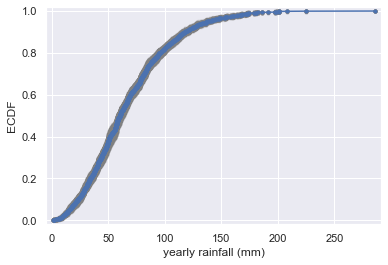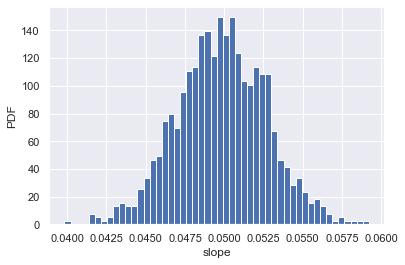import numpy as np
import pandas as pd
import matplotlib.pyplot as plt
import seaborn as sns

sns.set()

def ecdf(data):
"""Compute ECDF for a one-dimensional array of measurements."""
# Number of data points: n
n = len(data)

# x-data for the ECDF: x
x = np.sort(data)

# y-data for the ECDF: y
y = np.arange(1, n + 1) / n

return x, y


## Generating bootstrap replicates

### Bootstrapping

• The use of resampled data to perform statistical inference
• Bootstrap sample : A resampled array of the data
• Bootstrap replicate : A statistic computed from a resampled array

### Visualizing bootstrap samples

In this exercise, you will generate bootstrap samples from the set of annual rainfall data measured at the Sheffield Weather Station in the UK from 1883 to 2015. The data are stored in the NumPy array rainfall in units of millimeters (mm). By graphically displaying the bootstrap samples with an ECDF, you can get a feel for how bootstrap sampling allows probabilistic descriptions of data.

df = pd.read_fwf('./dataset/sheffield_weather_station.csv', skiprows=8)
rainfall = df['rain'].astype('float')

for _ in range(50):
# Generate bootstrap samle: bs_sample
bs_sample = np.random.choice(rainfall, size=len(rainfall))

# Compute and plot ECDF from bootstrap sample
x, y = ecdf(bs_sample)
_ = plt.plot(x, y, marker='.', linestyle='none', color='gray', alpha=0.1)

# Compute and plot ECDF from original data
x, y = ecdf(rainfall)
_ = plt.plot(x, y, marker='.')

# Make margins and label axes
plt.margins(0.02)
_ = plt.xlabel('yearly rainfall (mm)')
_ = plt.ylabel('ECDF')## Bootstrap confidence intervals

• Confidence interval of a statistics
• If we repeated measurements over and over again, p% of the observed values would lie within the p% confidence interval

### Generating many bootstrap replicates

The function bootstrap_replicate_1d() from the video is available in your namespace. Now you'll write another function, draw_bs_reps(data, func, size=1), which generates many bootstrap replicates from the data set. This function will come in handy for you again and again as you compute confidence intervals and later when you do hypothesis tests.

For your reference, the bootstrap_replicate_1d() function is provided below:

def bootstrap_replicate_1d(data, func):
"""Generate bootstrap replicate of 1D data."""
bs_sample = np.random.choice(data, len(data))
return func(bs_sample)

def bootstrap_replicate_1d(data, func):
"""Generate bootstrap replicate of 1D data."""
bs_sample = np.random.choice(data, len(data))
return func(bs_sample)

def draw_bs_reps(data, func, size=1):
"""Draw bootstrap replicates."""

# Initialize array of replicates: bs_replicates
bs_replicates = np.empty(size)

# Generate replicates
for i in range(size):
bs_replicates[i] = bootstrap_replicate_1d(data, func)

return bs_replicates


### Bootstrap replicates of the mean and the SEM

In this exercise, you will compute a bootstrap estimate of the probability density function of the mean annual rainfall at the Sheffield Weather Station. Remember, we are estimating the mean annual rainfall we would get if the Sheffield Weather Station could repeat all of the measurements from 1883 to 2015 over and over again. This is a probabilistic estimate of the mean. You will plot the PDF as a histogram, and you will see that it is Normal.

In fact, it can be shown theoretically that under not-too-restrictive conditions, the value of the mean will always be Normally distributed. (This does not hold in general, just for the mean and a few other statistics.) The standard deviation of this distribution, called the standard error of the mean, or SEM, is given by the standard deviation of the data divided by the square root of the number of data points. I.e., for a data set, sem = np.std(data) / np.sqrt(len(data)). Using hacker statistics, you get this same result without the need to derive it, but you will verify this result from your bootstrap replicates.

bs_replicates = draw_bs_reps(rainfall, np.mean, size=10000)

# Compute and print SEM
sem = np.std(rainfall) / np.sqrt(len(rainfall))
print(sem)

# Compute and print standard deviation of bootstrap replicates
bs_std = np.std(bs_replicates)
print(bs_std)

# Make a histogram of the results
_ = plt.hist(bs_replicates, bins=50, density=True)
_ = plt.xlabel('mean annual rainfall (mm)')
_ = plt.ylabel('PDF')

0.9488593574676786
0.956034708235844### Confidence intervals of rainfall data

A confidence interval gives upper and lower bounds on the range of parameter values you might expect to get if we repeat our measurements. For named distributions, you can compute them analytically or look them up, but one of the many beautiful properties of the bootstrap method is that you can take percentiles of your bootstrap replicates to get your confidence interval. Conveniently, you can use the np.percentile() function.

Use the bootstrap replicates you just generated to compute the 95% confidence interval. That is, give the 2.5th and 97.5th percentile of your bootstrap replicates stored as bs_replicates. What is the 95% confidence interval?

np.percentile(bs_replicates, [2.5, 97.5])

array([64.86782928, 68.62363296])

### Bootstrap replicates of other statistics

We saw in a previous exercise that the mean is Normally distributed. This does not necessarily hold for other statistics, but no worry: as hackers, we can always take bootstrap replicates! In this exercise, you'll generate bootstrap replicates for the variance of the annual rainfall at the Sheffield Weather Station and plot the histogram of the replicates.

Here, you will make use of the draw_bs_reps() function you defined a few exercises ago.

bs_replicates = draw_bs_reps(rainfall, np.var, size=10000)

# Put the variance in units of square centimeters
bs_replicates /= 100

# Make a histogram of the results
_ = plt.hist(bs_replicates, bins=50, density=True)
_ = plt.xlabel('variance of annual rainfall (sq. cm)')
_ = plt.ylabel('PDF')### Confidence interval on the rate of no-hitters

Consider again the inter-no-hitter intervals for the modern era of baseball. Generate 10,000 bootstrap replicates of the optimal parameter $\tau$. Plot a histogram of your replicates and report a 95% confidence interval.

nohitter_times = np.array([ 843, 1613, 1101,  215,  684,  814,  278,  324,  161,  219,  545,
715,  966,  624,   29,  450,  107,   20,   91, 1325,  124, 1468,
104, 1309,  429,   62, 1878, 1104,  123,  251,   93,  188,  983,
166,   96,  702,   23,  524,   26,  299,   59,   39,   12,    2,
308, 1114,  813,  887,  645, 2088,   42, 2090,   11,  886, 1665,
1084, 2900, 2432,  750, 4021, 1070, 1765, 1322,   26,  548, 1525,
77, 2181, 2752,  127, 2147,  211,   41, 1575,  151,  479,  697,
557, 2267,  542,  392,   73,  603,  233,  255,  528,  397, 1529,
1023, 1194,  462,  583,   37,  943,  996,  480, 1497,  717,  224,
219, 1531,  498,   44,  288,  267,  600,   52,  269, 1086,  386,
176, 2199,  216,   54,  675, 1243,  463,  650,  171,  327,  110,
774,  509,    8,  197,  136,   12, 1124,   64,  380,  811,  232,
192,  731,  715,  226,  605,  539, 1491,  323,  240,  179,  702,
156,   82, 1397,  354,  778,  603, 1001,  385,  986,  203,  149,
576,  445,  180, 1403,  252,  675, 1351, 2983, 1568,   45,  899,
3260, 1025,   31,  100, 2055, 4043,   79,  238, 3931, 2351,  595,
110,  215,    0,  563,  206,  660,  242,  577,  179,  157,  192,
192, 1848,  792, 1693,   55,  388,  225, 1134, 1172, 1555,   31,
1582, 1044,  378, 1687, 2915,  280,  765, 2819,  511, 1521,  745,
2491,  580, 2072, 6450,  578,  745, 1075, 1103, 1549, 1520,  138,
1202,  296,  277,  351,  391,  950,  459,   62, 1056, 1128,  139,
420,   87,   71,  814,  603, 1349,  162, 1027,  783,  326,  101,
876,  381,  905,  156,  419,  239,  119,  129,  467])

bs_replicates = draw_bs_reps(nohitter_times, np.mean, 10000)

# Compute the 95% confidence interval: conf_int
conf_int = np.percentile(bs_replicates, [2.5, 97.5])

# Print the confidence interval
print('95% confidence interval =', conf_int, 'games')

# Plot the histogram of the replicates
_ = plt.hist(bs_replicates, bins=50, density=True)
_ = plt.xlabel(r'$\tau$ (games)')
_ = plt.ylabel('PDF')

95% confidence interval = [663.32300797 871.85756972] games## Pairs bootstrap

• Nonparametric inference
• Make no assumptions about the model or probability distribution underlying the data
• Pairs bootstrap for linear regression
• Resample data in pairs
• Compute slope and intercept from resampled data
• Each slope and intercept is a bootstrap replicate

### A function to do pairs bootstrap

As discussed in the video, pairs bootstrap involves resampling pairs of data. Each collection of pairs fit with a line, in this case using np.polyfit(). We do this again and again, getting bootstrap replicates of the parameter values. To have a useful tool for doing pairs bootstrap, you will write a function to perform pairs bootstrap on a set of x,y data.

def draw_bs_pairs_linreg(x, y, size=1):
"""Perform pairs bootstrap for linear regression."""

# Set up array of indices to sample from: inds
inds = np.arange(len(x))

# Initialize replicates: bs_slope_reps, bs_intercept_reps
bs_slope_reps = np.empty(size)
bs_intercept_reps = np.empty(size)

# Generate replicates
for i in range(size):
bs_inds = np.random.choice(inds, size=len(inds))
bs_x, bs_y = x[bs_inds], y[bs_inds]
bs_slope_reps[i], bs_intercept_reps[i] = np.polyfit(bs_x, bs_y, deg=1)

return bs_slope_reps, bs_intercept_reps


### Pairs bootstrap of literacy/fertility data

Using the function you just wrote, perform pairs bootstrap to plot a histogram describing the estimate of the slope from the illiteracy/fertility data. Also report the 95% confidence interval of the slope. The data is available to you in the NumPy arrays illiteracy and fertility.

As a reminder, draw_bs_pairs_linreg() has a function signature of draw_bs_pairs_linreg(x, y, size=1), and it returns two values: bs_slope_reps and bs_intercept_reps.

df = pd.read_csv('./dataset/female_literacy_fertility.csv')
fertility = np.array(df['fertility'])
illiteracy = np.array(100 - df['female literacy'])

bs_slope_reps, bs_intercept_reps = draw_bs_pairs_linreg(illiteracy, fertility, 1000)

# Compute and print 95% CI for slope
print(np.percentile(bs_slope_reps, [2.5, 97.5]))

# Plot the histogram
_ = plt.hist(bs_slope_reps, bins=50, density=True)
_ = plt.xlabel('slope')
_ = plt.ylabel('PDF')

[0.04409522 0.05545012]### Plotting bootstrap regressions

A nice way to visualize the variability we might expect in a linear regression is to plot the line you would get from each bootstrap replicate of the slope and intercept. Do this for the first 100 of your bootstrap replicates of the slope and intercept.

x = np.array([0, 100])

# Plot the bootstrap lines
for i in range(100):
_ = plt.plot(x,
bs_slope_reps[i] * x + bs_intercept_reps[i],
linewidth=0.5, alpha=0.2, color='red')

# Plot the data
_ = plt.plot(illiteracy, fertility, marker='.', linestyle='none')

# Label axes, set the margins, and show the plot
_ = plt.xlabel('illiteracy')
_ = plt.ylabel('fertility')
plt.margins(0.02)
plt.savefig('../images/bootstrap-reg.png')